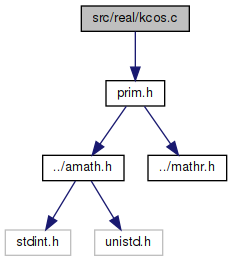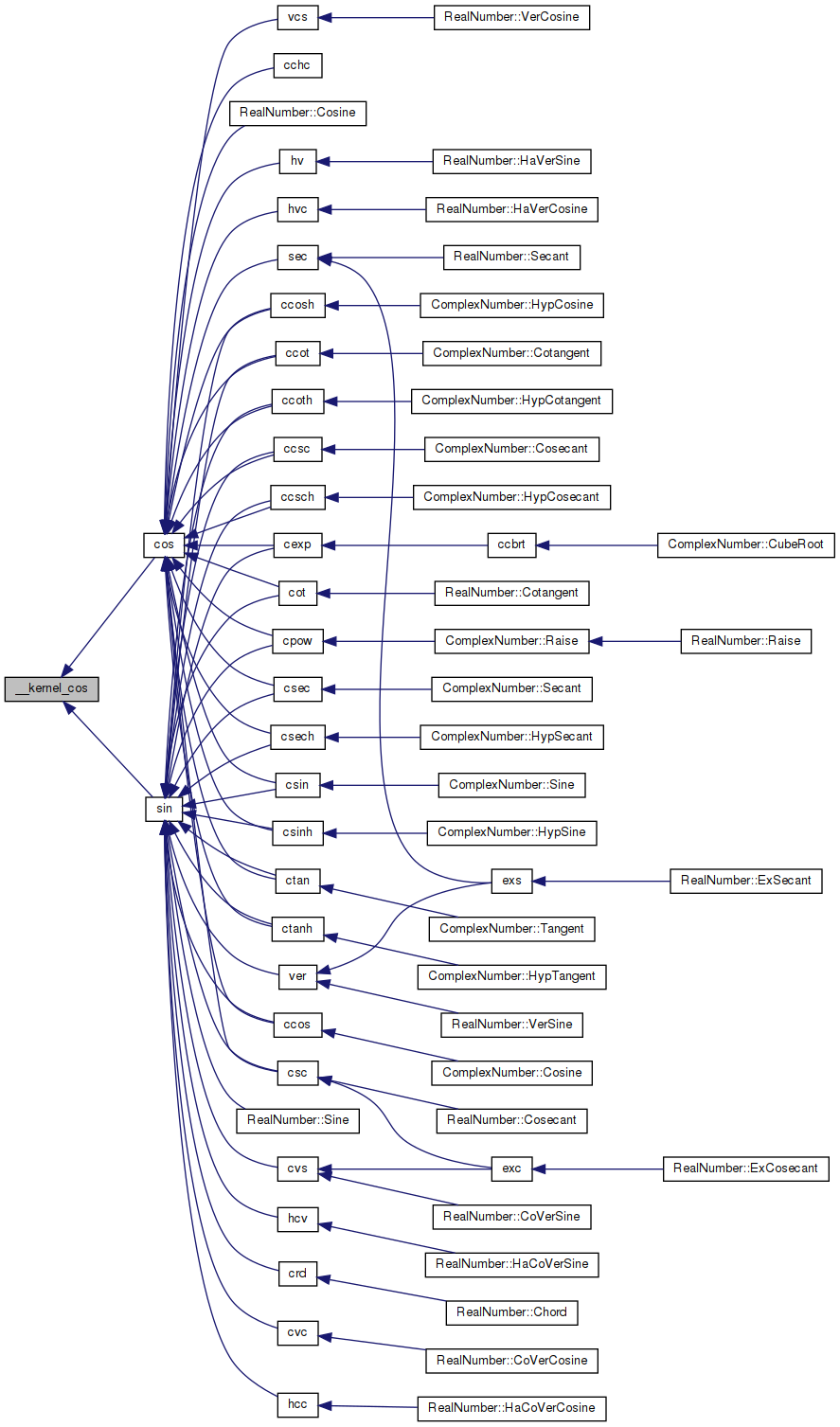amath  1.8.5 Simple command line calculator
kcos.c File Reference

Kernel cosine function. More...

`#include "prim.h"`
Include dependency graph for kcos.c:Go to the source code of this file.

## Functions

double __kernel_cos (double x, double y)
Kernel cosine function. More...

## Variables

static const double one = 1.00000000000000000000e+00

static const double C1 = 4.16666666666666019037e-02

static const double C2 = -1.38888888888741095749e-03

static const double C3 = 2.48015872894767294178e-05

static const double C4 = -2.75573143513906633035e-07

static const double C5 = 2.08757232129817482790e-09

static const double C6 = -1.13596475577881948265e-11

## Detailed Description

Kernel cosine function.

Definition in file kcos.c.

## ◆ __kernel_cos()

 double __kernel_cos ( double x, double y )

Kernel cosine function.

```Kernel cos function on [-pi/4, pi/4], pi/4 ~ 0.785398164
Input x is assumed to be bounded by ~pi/4 in magnitude.
Input y is the tail of x.```
```Algorithm
1. Since cos(-x) = cos(x), we need only to consider positive x.
2. if x < 2^-27 (hx<0x3e400000 0), return 1 with inexact if x!=0.
3. cos(x) is approximated by a polynomial of degree 14 on
[0,pi/4]
4            14
cos(x) ~ 1 - x*x/2 + C1*x + ... + C6*x
where the Remes error is```
```    |              2     4     6     8     10    12     14 |     -58
|cos(x)-(1-.5*x +C1*x +C2*x +C3*x +C4*x +C5*x  +C6*x  )| <= 2
|                                      |
4     6     8     10    12     14

4. let r = C1*x +C2*x +C3*x +C4*x +C5*x  +C6*x  , then
cos(x) = 1 - x*x/2 + r
since cos(x+y) ~ cos(x) - sin(x)*y
~ cos(x) - x*y,
a correction term is necessary in cos(x) and hence
cos(x+y) = 1 - (x*x/2 - (r - x*y))
For better accuracy when x > 0.3, let qx = |x|/4 with
the last 32 bits mask off, and if x > 0.78125, let qx = 0.28125.
Then
cos(x+y) = (1-qx) - ((x*x/2-qx) - (r-x*y)).
Note that 1-qx and (x*x/2-qx) is EXACT here, and the
magnitude of the latter is at least a quarter of x*x/2,
thus, reducing the rounding error in the subtraction.
```

Definition at line 94 of file kcos.c.

References C1, C2, C3, C4, C5, C6, and one.

Referenced by cos(), and sin().

95 {
96  double a, hz, z, r, qx;
97  int32_t ix;
98
99  GET_HIGH_WORD(ix, x);
100  ix &= 0x7FFFFFFF;
101
102  // if x < 2**27
103  if (ix < 0x3E400000)
104  {
105  // generate inexact
106  if ((int)x == 0)
107  {
108  return one;
109  }
110  }
111
112  z = x * x;
113  r = z * (C1 + z * (C2 + z * (C3 + z * (C4 + z * (C5 + z * C6)))));
114
115  // |x| < 0.3
116  if (ix < 0x3FD33333)
117  {
118  return one - (0.5 * z - (z * r - x * y));
119  }
120
121  // x > 0.78125
122  if (ix > 0x3FE90000)
123  {
124  qx = 0.28125;
125  }
126  else
127  {
128  INSERT_WORDS(qx, ix - 0x00200000, 0);
129  }
130
131  hz = 0.5 * z - qx;
132  a = one - qx;
133  return a - (hz - (z * r - x * y));
134 }
#define INSERT_WORDS(d, ix0, ix1)
Set a double from two 32 bit ints.
Definition: prim.h:187
#define GET_HIGH_WORD(i, d)
Get the more significant 32 bit int from a double.
Definition: prim.h:167
static const double C1
Definition: kcos.c:50
static const double C5
Definition: kcos.c:54
static const double C6
Definition: kcos.c:55
static const double C3
Definition: kcos.c:52
static const double one
Definition: kcos.c:49
static const double C4
Definition: kcos.c:53
static const double C2
Definition: kcos.c:51
Here is the caller graph for this function:## ◆ C1

 const double C1 = 4.16666666666666019037e-02
static

Definition at line 50 of file kcos.c.

Referenced by __kernel_cos().

## ◆ C2

 const double C2 = -1.38888888888741095749e-03
static

Definition at line 51 of file kcos.c.

Referenced by __kernel_cos().

## ◆ C3

 const double C3 = 2.48015872894767294178e-05
static

Definition at line 52 of file kcos.c.

Referenced by __kernel_cos().

## ◆ C4

 const double C4 = -2.75573143513906633035e-07
static

Definition at line 53 of file kcos.c.

Referenced by __kernel_cos().

## ◆ C5

 const double C5 = 2.08757232129817482790e-09
static

Definition at line 54 of file kcos.c.

Referenced by __kernel_cos().

## ◆ C6

 const double C6 = -1.13596475577881948265e-11
static

Definition at line 55 of file kcos.c.

Referenced by __kernel_cos().

## ◆ one

 const double one = 1.00000000000000000000e+00
static

Definition at line 49 of file kcos.c.

Referenced by __kernel_cos().